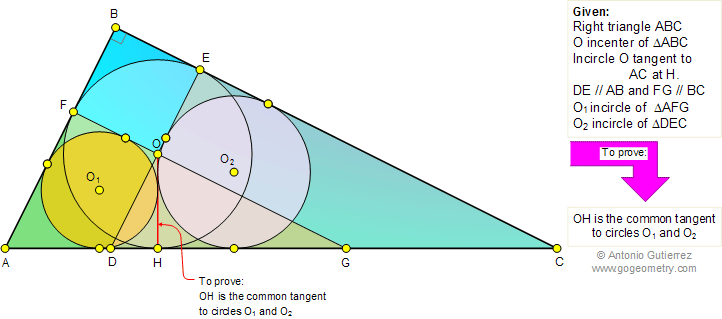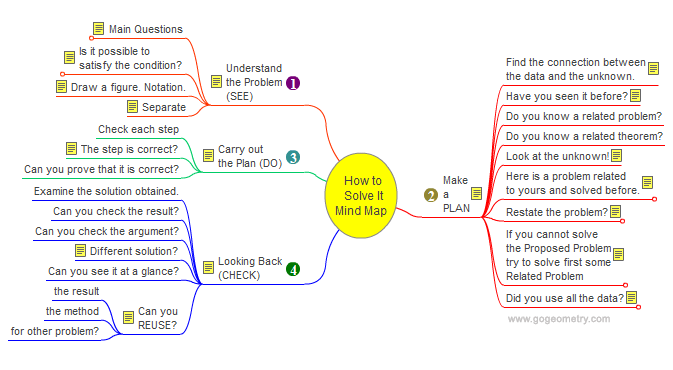# Problem 668: Right triangle, Incircles, Parallel, Common Tangent, Mind Map## Level: High School, Honors Geometry, College Geometry

 The figure shows a right triangle ABC with the incircle O tangent to AC at H. From O parallels DE and FG are drawn to AB, and BC, respectively. Prove that OH is the common tangent to the incircles of triangles AFG and DEC.Geometry problem solving Geometry problem solving is one of the most challenging skills for students to learn. When a problem requires auxiliary construction, the difficulty of the problem increases drastically, perhaps because deciding which construction to make is an ill-structured problem. By “construction,” we mean adding geometric figures (points, lines, planes) to a problem figure that wasn’t mentioned as "given."   How to Solve It, Interactive Mind Map George Pólya's 1945 book "How to Solve It, A new aspect of Mathematical Method", is a book describing methods of problem solving. It suggests the following steps when solving a mathematical problem: (1) First, you have to understand the problem. (2) After understanding, then make a plan. (3) Carry out the plan. (4) Look back on your work. How could it be better?    Mind Map Help. To see a note: Hover over a yellow note button. To Fold/Unfold: click a branch. To Pan: click and drag the map canvas.Home | Search | Geometry | Problems | All Problems | Open Problems | Visual Index | 661-670 | Circles | Right Triangle | Square | Incenter/Incircle | Email | Post a comment or solution | By Antonio Gutierrez